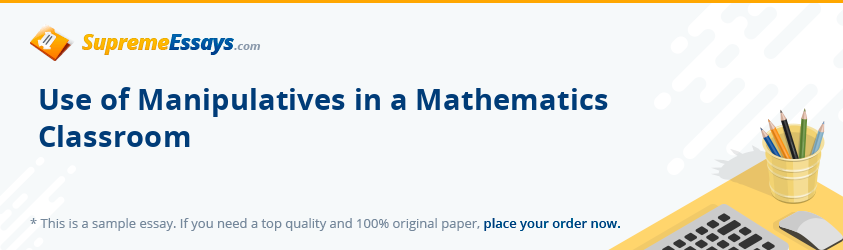Live chatNot all students in a classroom get every concept during the first lesson by a teacher, especially the mathematical concepts. It is even worse if the teacher does not use concrete objects to illustrate the said concepts. Concrete objects that aid in teaching get referred to as manipulatives, and if used effectively, they greatly enhance teaching of mathematical aspects of the curriculum.

According to research, manipulative materials make understanding of mathematics by students more effective. An object or material that children use to show and comprehend mathematical concepts is referred to as mathematical manipulatives. These objects can appeal to the senses and are tangible, concrete hands-on model that the students can use to understand effectively various mathematical concepts (Tammie, 2011). Such objects connect to a student’s real world. Classrooms have become a commonplace for manipulatives such as buttons and counting sticks. These enable students to be taught a concept actively by their teachers. If they are to use manipulatives successfully   in teaching mathematics, teachers have to know how to use them beforehand. The failures that have been reported in the use of manipulatives have resulted from teachers not knowing how to use the objects themselves (Hudson, 2007).

Manipulatives can be used as a remedial for those students who fail to get a concept even after a teacher explains it a number of times. They come in handy when re teaching a concept, as they greatly enhance a student’s understanding of that concept. Various manipulatives are used in teaching mathematics. Students easily learn geometric shapes, through counting and feeling the edges of cardboard and design shapes. Fractions are among the most difficult mathematical aspects for most students. Through coloring and cutting part of a whole concrete object, students understand fractions without many problems.

0

Preparing Orders

0

Active Writers

0

Support Agents